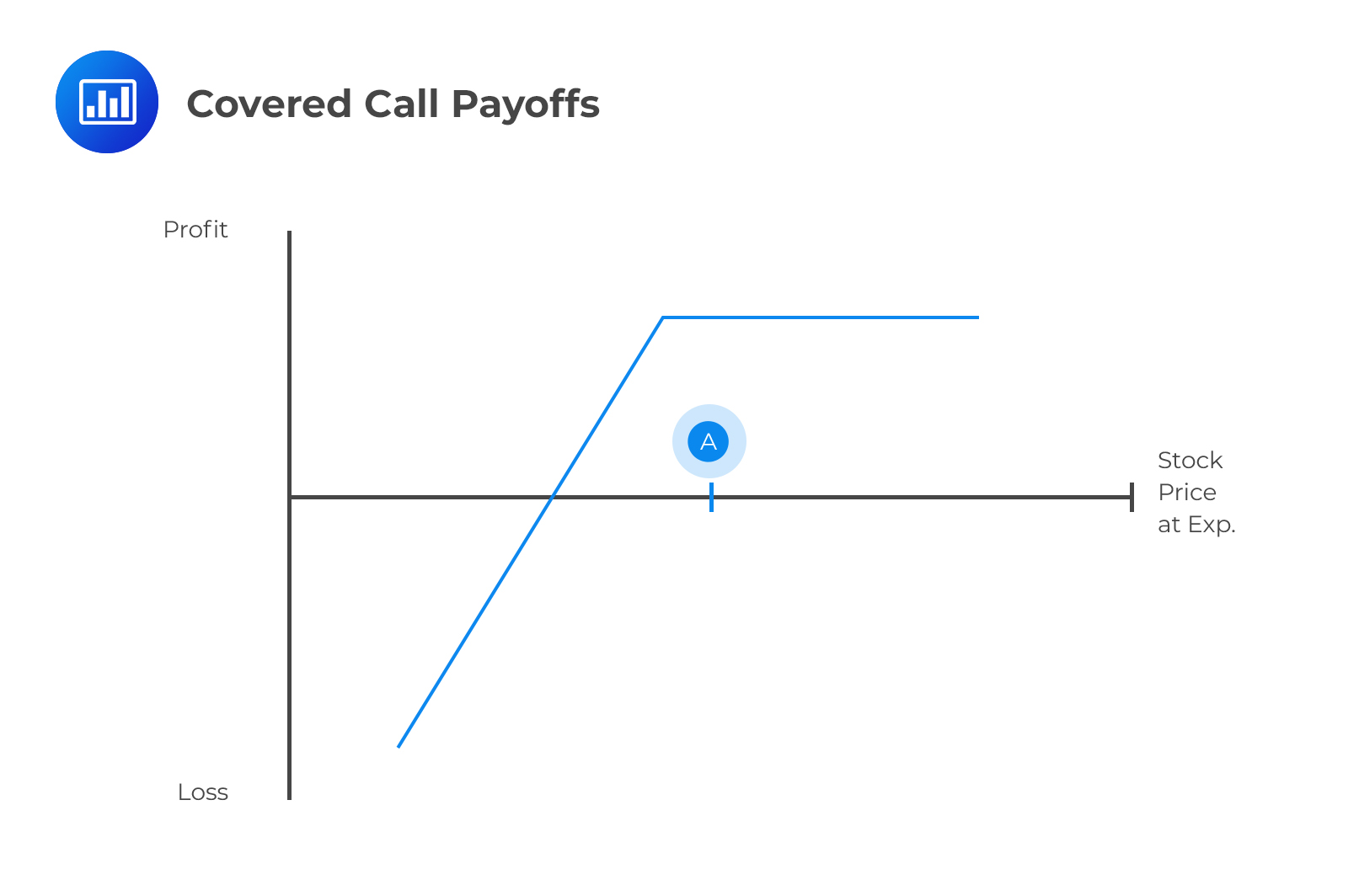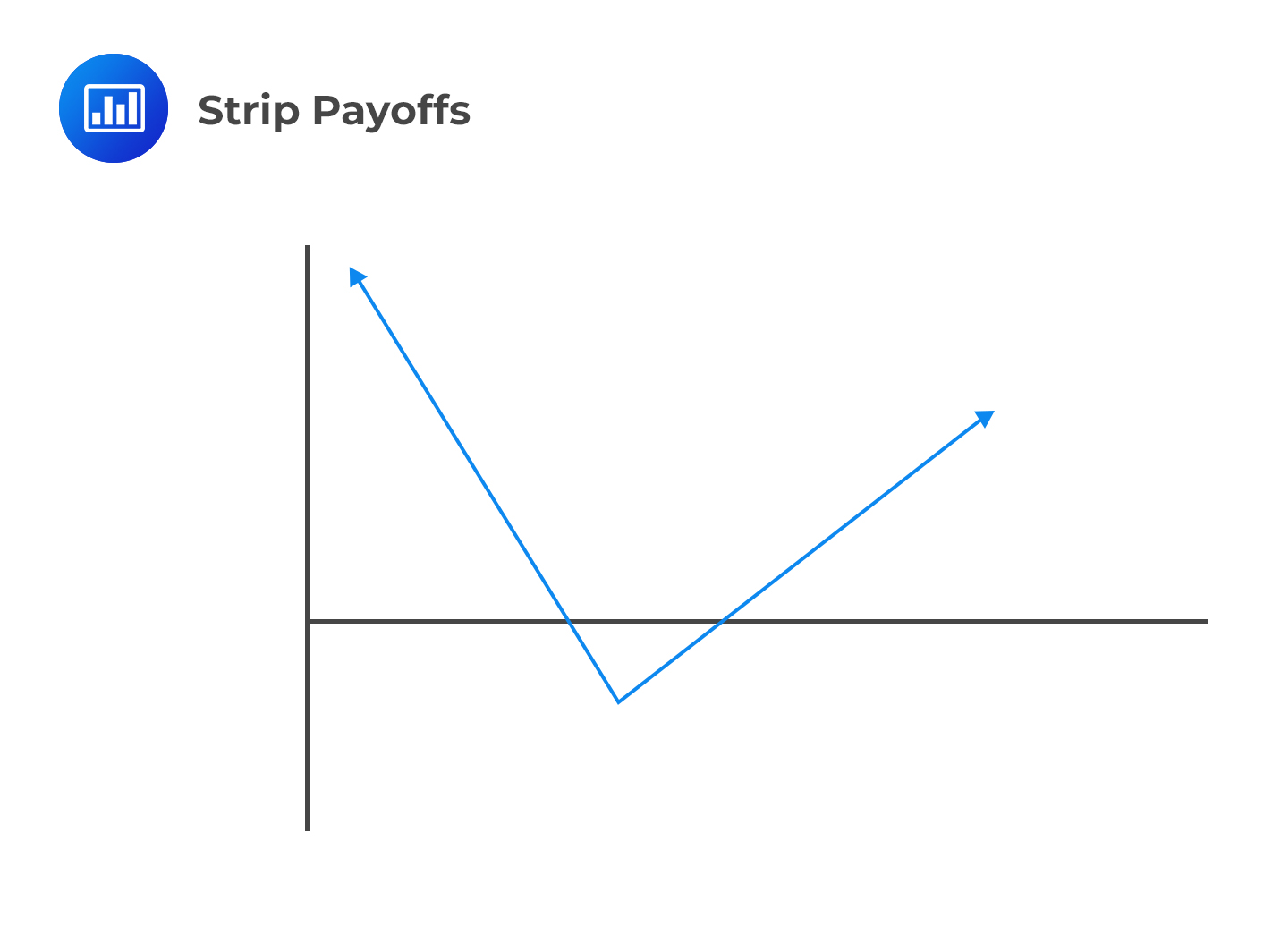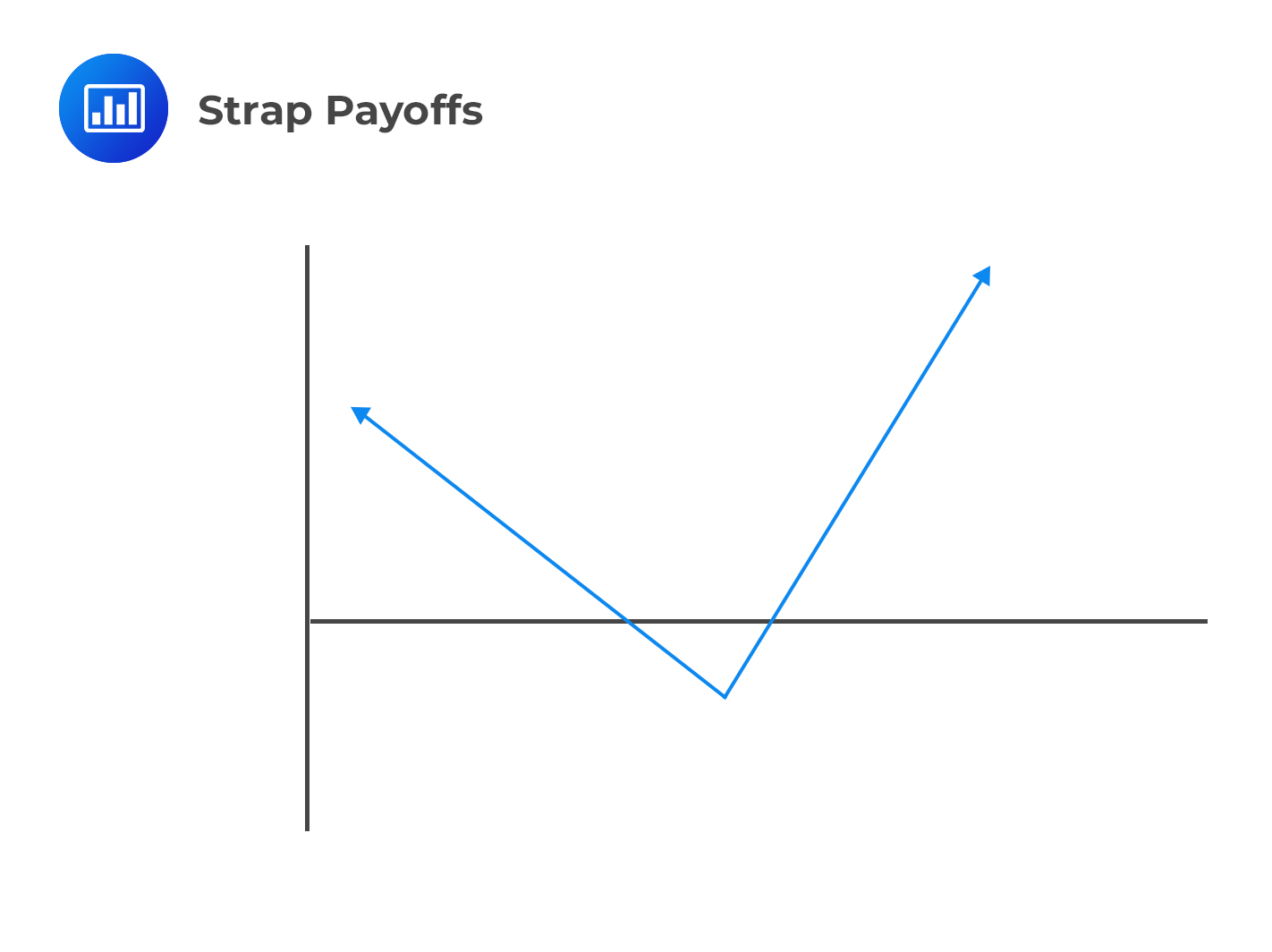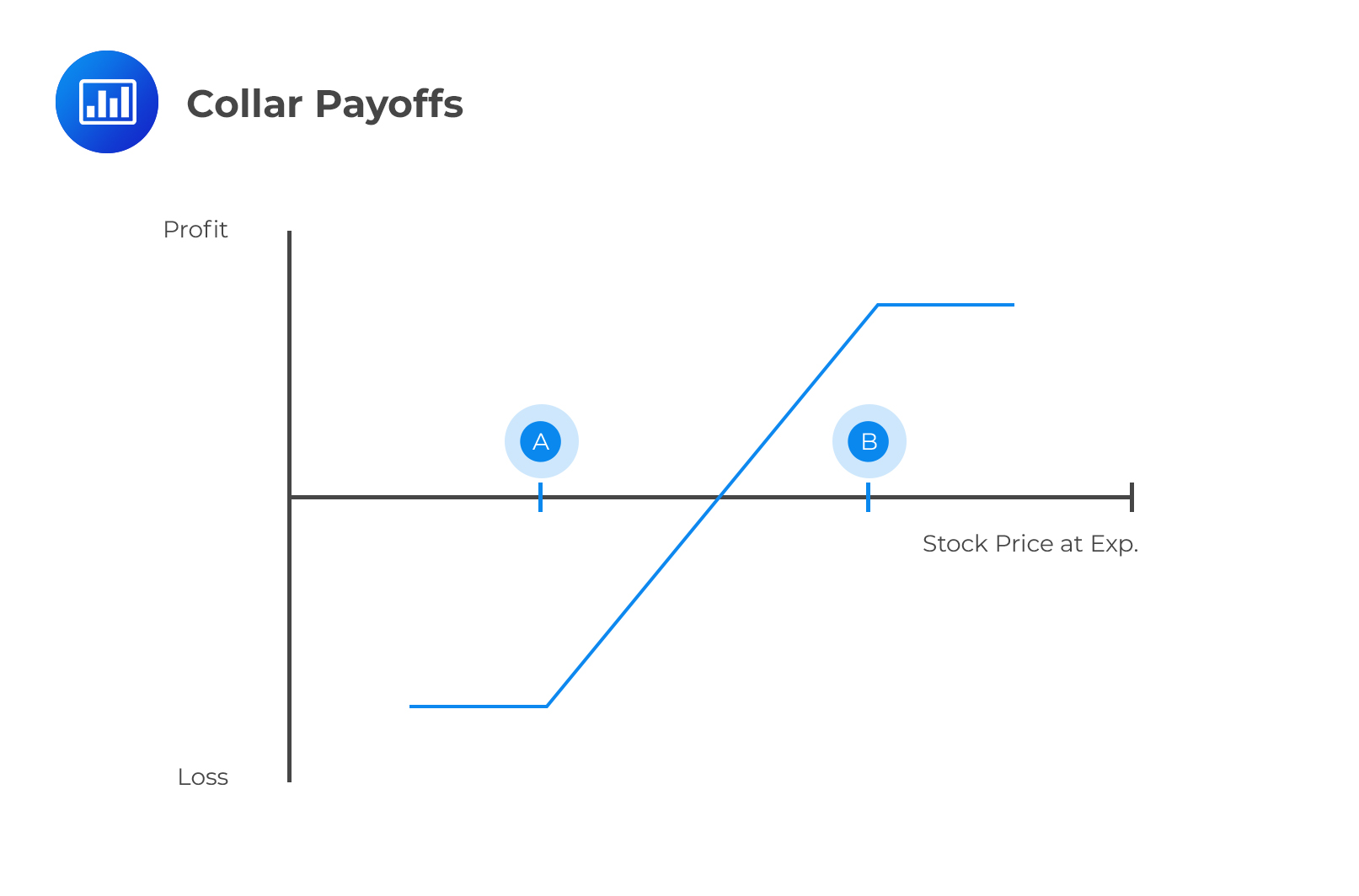Limited Time Offer: Save 10% on all 2022 Premium Study Packages with promo code: BLOG10After completing this reading, you should be able to:

• Explain the motivation to initiate a covered call or a protective put strategy.
• Describe principal-protected notes (PPNs) and explain necessary conditions to create a PPN.
• Describe the use and calculate the payoffs of various spread strategies.
• Describe the use and explain the payoff functions of combination strategies.

Options can be arranged in different ways to form different payoff patterns.
Strategies that involve a single option are referred to as spreads, while those involving both call and put options are referred to as combinations.

## Strategies Involving a Single Option

In the previous chapter, we looked at the put-call parity relationship, which is given by:

$$c + PV(K)= p + S_0………….(i)$$
Where:
$$c =$$ value of call option
$$K =$$ strike price
$$p =$$ value of put option
$$S_0=$$ initial stock price
$$r =$$ risk-free rate
$$T =$$time to maturity
$$PV(K) =$$present value of the strike price

The above result can also be expressed as:
$$S_0-c=PV(K)-p$$……………(ii)
$$-p -S_0=-c-PV(K)$$………(iii)
$$c -S_0=p-PV(K)$$…………(iv)
The right-hand side of equation (i) shows a combination of a put and an asset.
When you buy a put while holding the underlying asset, it is referred to as a protective put strategy.
The left-hand side of equation (ii) shows an asset plus a short call. This strategy is referred to as a covered call.
Equation iii shows the reverse of a protected put, while equation iv illustrates the reverse of a covered call.

## Covered Call

A covered call describes a trading strategy where the seller (writer) of a call option also owns the underlying stock. The writer sells call options for the same amount (or less) of stock. If the option is not exercised, the writer gets to keep the premium. If the option is exercised, the writer simply hands the option buyer their shares. In a covered call, the important thing to note is that the investor receives a premium in exchange for giving up any potential profits from high upward movements of the asset price.

A covered call is the opposite of a naked call. In the latter, the writer of the call option does not own the underlying stock. However, in the event that the option is exercised, the writer is obligated to buy the shares at the market price and deliver them to the option buyer. A naked call, therefore, has an unlimited risk because the market price can rise unpredictably.

The holder of a covered call can only profit on the stock up to the strike price of the options contract. The maximum profit is capped at:

$$\text{(Strike Price – Stock Entry Price) + Option Premium Received}$$For example, let’s say you’ve bought a stock at $10, received a$0.50 option premium from selling a $12 strike price call. You’d maintain your stock position as long as the stock price stays below$12 at expiration. If the stock rises to $$13$$, you will only profit up to $12, so your total profit will be $$2.5\left( =12-10+0.50 \right)$$. You’d be forced to give up the extra$0.50.

The maximum loss a covered call holder can incur is equal to:

$$\text{Stock Entry Price}-0+\text{Option Premium Received}$$

3. ##### Strips:

A strip is literally a long straddle, but the only difference is that a strip involves the purchase of two puts and one call(instead of one each as in straddle) with the same strike price and expiration. An investor enters into a long strip position when he expects a large move in a stock and considers a decrease in the stock price more likely than an increase.4. ##### Straps:

A long strap is a mirror image of a strip strategy. It consists of a long position in two calls and one put with the same exercise price and expiration date. An investor enters into a long strap position when he expects large moves in a stock but considers an increase in the stock price more likely than a decrease.5. Collar: A collar is a combination of a protective put and a covered call.## Interest Rate Caps and Floors

An interest rate cap is a type of interest rate derivative in which the buyer receives payments at the end of each period in which the interest rate exceeds the agreed strike price. The strike rate is also called the cap rate. For example, the buyer could receive payments when the rate exceeds LIBOR + 200bps.

On the other hand, an interest rate floor is a derivative contract in which the buyer receives payments at the end of periods in which the interest rate is below the agreed strike price. For example, the buyer could be entitled to a payment whenever the rate is below LIBOR + 50 bps.

Interest rate caps and floors provide protection against fluctuating interest rates.

## Practice Questions

### Question 1

Which of the following options trading strategies does not expose the party to unlimited losses?

A. Naked call

B. Covered call

C. Short strangle

Options strategies that expose the party to unlimited losses include:

• Covered call
• Short strangle etc.

### Question 2

A trader wants to create a long strangle trading strategy with a strike prices of $50 for the call option and$40 for the put option. The prices of four-month European call and put options are $3.12 and$2.87, respectively. At maturity, the stock price has to be greater than which of the following to realize profits?

A. $50 B.$34.01

C. $53.12 D.$55.99

The question is testing on the use of the long strangle trading strategy, a trading strategy that involves buying a call option with the higher strike price, and buying a put option with the lower strike price.

The strike price for the call (shown as B on the graph) = $50 The strike price for the put (shown as A on the graph) =$40

Cost of setting up the long strangle = $3.12 +$2.87 = $5.99 Upper price bound of the asset price at maturity =$50 + $5.99 =$55.99

Lower price bound of the asset price at maturity = $40 –$5.99 = \$34.01

Featured Study with UsCFA® Exam and FRM® Exam Prep Platform offered by AnalystPrep

Study Platform

Learn with Us

Subscribe to our newsletter and keep up with the latest and greatest tips for success
Online TutoringOur videos feature professional educators presenting in-depth explanations of all topics introduced in the curriculum.

Video LessonsDaniel Glyn
2021-03-24
I have finished my FRM1 thanks to AnalystPrep. And now using AnalystPrep for my FRM2 preparation. Professor Forjan is brilliant. He gives such good explanations and analogies. And more than anything makes learning fun. A big thank you to Analystprep and Professor Forjan. 5 stars all the way!michael walshe
2021-03-18
Professor James' videos are excellent for understanding the underlying theories behind financial engineering / financial analysis. The AnalystPrep videos were better than any of the others that I searched through on YouTube for providing a clear explanation of some concepts, such as Portfolio theory, CAPM, and Arbitrage Pricing theory. Watching these cleared up many of the unclarities I had in my head. Highly recommended.Nyka Smith
2021-02-18
Every concept is very well explained by Nilay Arun. kudos to you man!2021-02-13